Open in App
Not now

# Class 10 RD Sharma Solutions – Chapter 10 Circles – Exercise 10.2 | Set 1

• Last Updated : 05 May, 2021

### Question 1. If PT is a tangent at T to a circle whose centre is O and OP = 17 cm, OT = 8 cm. Find the length of the tangent segment PT.

Solution: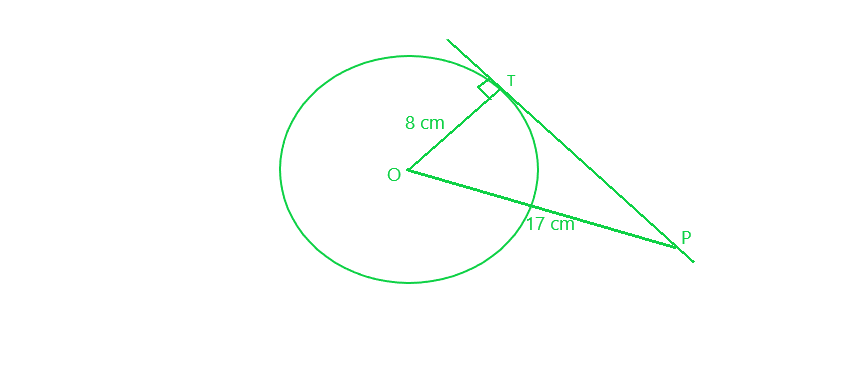PT is the tangent to the circle with centre O, at T

=> PT⊥ OT

OP = 17 cm

Therefore, In right ∆OPT, by applying Pythagoras Theorem:

OP² = OT² + PT²

=> (17)² = (8)² + PT²

=> 289 = 64 + PT²

=> PT² = 289 – 64 = 225 = (15)²

PT = 15 cm

### Question 2. Find the length of a tangent drawn to a circle with radius 5 cm, from a point 13 cm from the centre of the circle.

Solution: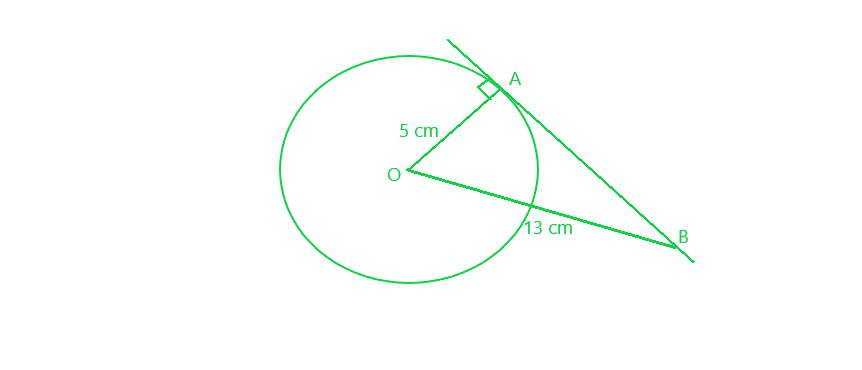From a point P outside the circle with centre O, let us draw a tangent PT to the circle

OB = 13 cm

OA ⊥ BA

Therefore, In right ∆OPT, by applying Pythagoras Theorem

OB² = OA² + BA²

(13)² = (5)² + BA²

=> 169 = 25 + BA²

=> BA² = 169 – 25 = 144 = (12)²

BA = 12 cm

### Question 3. A point P is 26 cm away from the centre O of a circle and the length PT of the tangent drawn from P to the circle is 10 cm. Find the radius of the circle.

Solution: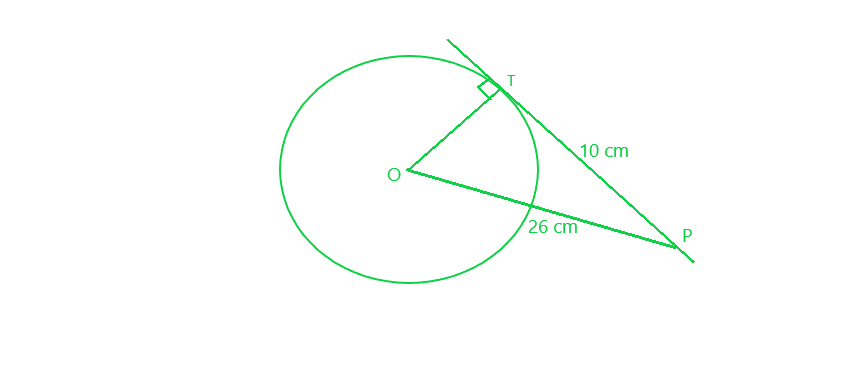From a point P outside the circle with centre O, let us draw a tangent PT to the circle of radius OT

OP = 26 cm

PT = 10 cm

Therefore, In right ∆OPT, by applying Pythagoras Theorem:

OP² = OT² + PT²

=> (26)² = OT² + (10)²

=> 676 = OT² + 100

=> 676 – 100 = OT²

=> OT² = 576 = (24)²

Hence, radius of the circle = 24 cm

### Question 4. If from any point on the common chord of two intersecting circles, tangents be drawn to the circles, prove that they are equal.

Solution: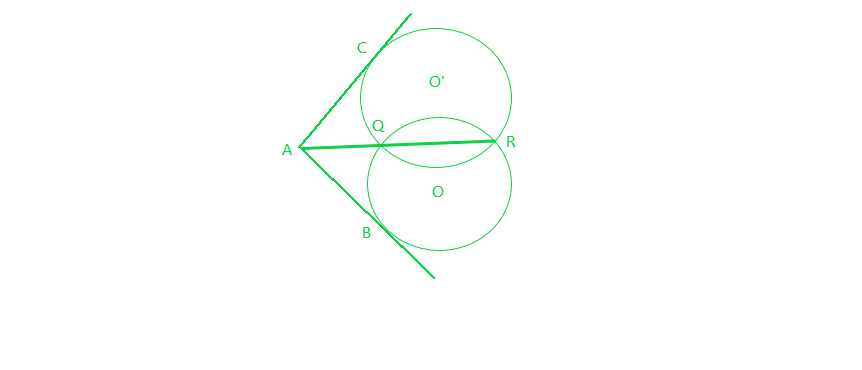Given:

QR is the common chord of two circles intersecting each other at Q and R

A is a point on RQ when produced.

From A, AB and AC two tangents are drawn to the circles with centres O and O’ respectively.

To prove : AB = AC

Proof: AB is the tangent and AQR is the secant to the circle with centre O

=> AB² = AQ x AR     (1)

Similarly, AC is the tangent and AQR is the secant to the circle with centre O’

=> AC² = AQ x AR     (2)

From (1) and (2)

AB² = AC²

AB = AC

Hence, proved.

### Question 5. If the sides of a quadrilateral touch a circle, prove that the sum of a pair of opposite sides is equal to the sum of the other pair.

Solution: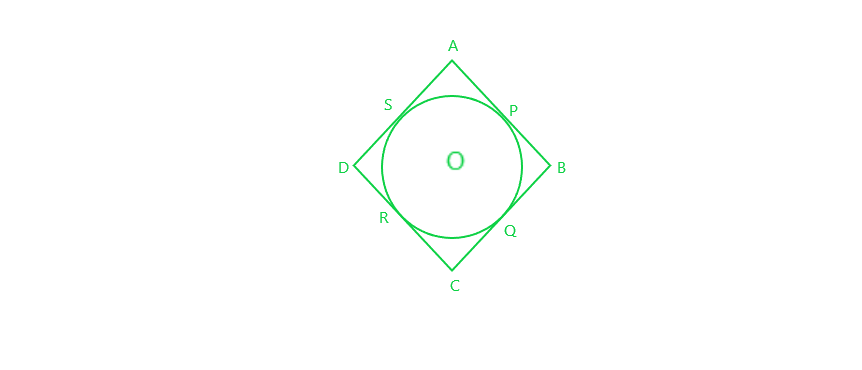Given: The sides of a quadrilateral ABCD touch the circle at P, Q, R and S respectively

To prove : AB + CD = AP + BC

Proof : AP and AS are the tangents to the circle from A

=> AP = AS     (1)

Similarly, BP = BQ     (2)

and CR = CQ     (3)

and DR = DS     (4)

Adding, all the four equation we get:

AP + BP + CR + DR = AS + BQ + CQ + DS

=> (AP + BP) + (CR + DR) = (AS + DS) + (BQ + CQ)

=> AB + CD = AD + BC

Hence, proved.

### Question 6. Out of the two concentric circles, the radius of the outer circle is 5 cm and the chord AC of length 8 cm is a tangent to the inner circle. Find the radius of the inner circle.

Solution: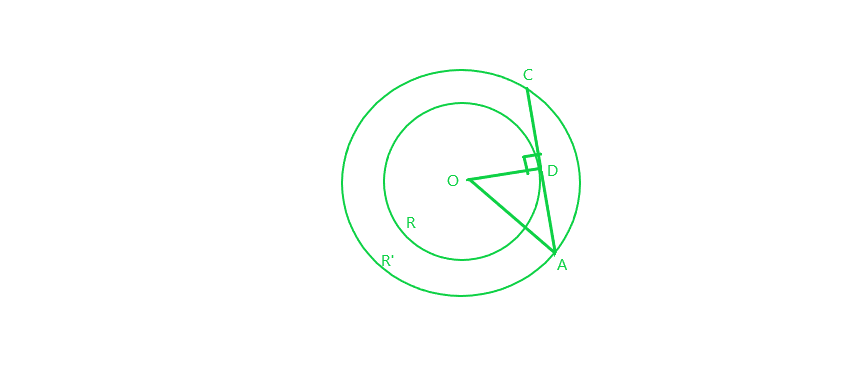Let R and R’ be the two circles having same centre O. AC is a chord which touches the R at point D

Now, OD ⊥ AC

AD = DC = 4 cm     [perpendicular line OD bisects the chord at D]

OA = 5 cm     [given]

Therefore, In right-angled ∆AOD, by applying Pythagoras theorem:

=> OD² = 5² – 4² = 25 – 16 = 9

=> OD = 3 cm

Thus, Radius of the inner circle OD = 3 cm

### Question 7. A chord PQ of a circle is parallel to the tangent drawn at a point R of the circle. Prove that R bisects the arc PRQ.

Solution:Given: Chord PQ is parallel to the tangent at R.

To prove : R bisects the arc PRQ (i.e., PR=QR)

Proof:

∠1 = ∠2       [alternate interior angles]   (1)

∠1 = ∠3      [angle between tangent and chord is equal to angle made by chord in alternate segment]   (2)

=> ∠2 = ∠3     [from (1) and (2)]

=> PR = QR      [sides opposite to equal angles are equal]

So, R bisects PQ

### Question 8. Prove that a diameter AB of a circle bisects all those chords which are parallel to the tangent at the point A.

Solution: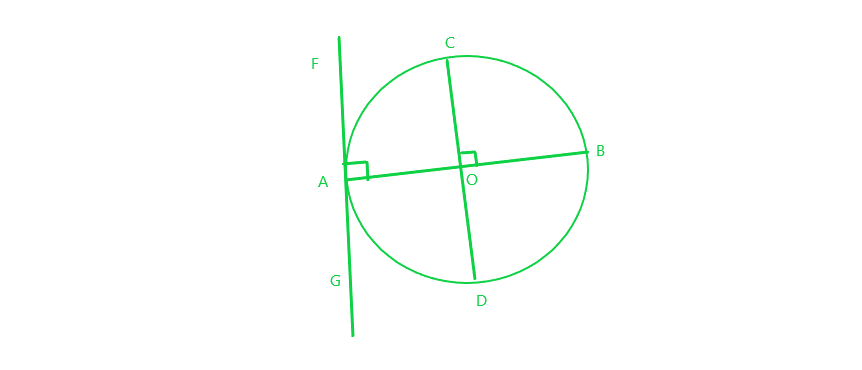Given:

AB is a diameter of the circle.

A tangent is drawn from point A.

A chord CD parallel to the tangent FAG.

To prove: AB bisect CD and hence all the chords parallel to the tangent at the point A.

Proof: CD is a chord of the circle and OA is a radius of the circle.

∠FAO = 90°     [Tangent at any point of a circle is perpendicular to the radius through the point of contact]

∠COB = ∠FAO      [corresponding angles]

=> ∠COB = 90°

Thus, AB bisects CD     [perpendicular from centre of circle to chord bisects the chord]

Similarly, the diameter AB bisects all the chords which are parallel to the tangent at the point A.

### Question 9. If AB, AC, PQ are the tangents in the figure, and AB = 5 cm, find the perimeter of ∆APQ.

Solution:AB, AC and PQ are the tangents to the circle as shown in the figure above and AB = 5 cm

PB and PR are the tangents to the circle from the same point P

=> PB = PR

Similarly, QC and QR are the tangents from the same point Q

=> QC = QR

Also, AB and AC are the tangents from A

=> AB = AC = 5 cm

Now perimeter of ∆APQ

= AP + PQ + AQ

= AP + PR + RQ + AQ

= AP + PB + QC + AQ      {because PB = PR and QC = QR}

= AB + AC

= 5 cm + 5 cm

=10 cm

So, Perimeter of the triangle APQ is 10 cm

### Question 10. Prove that the intercept of a tangent between two parallel tangents to a circle subtends a right angle at the centre.

Solution: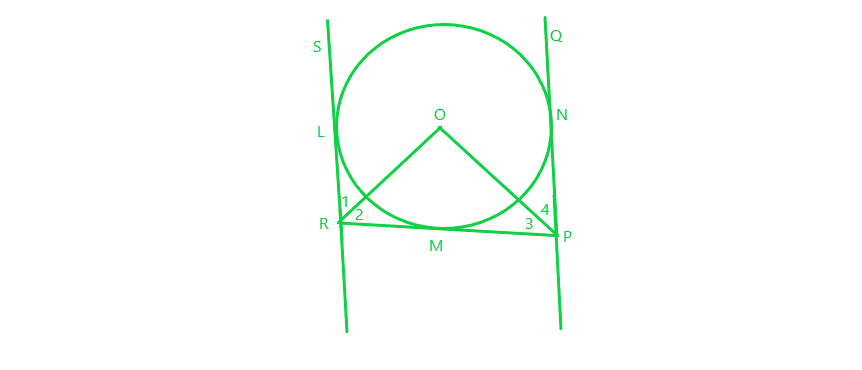Given:

PQ and RS are parallel tangents of a circle

RMP is the intercept of the tangent between PQ and RS

RS and PQ are joined at the centre of the circle O

To prove: ∠ROP = 90°

Proof:

Since RL and RM are tangents from R and RO is joined at the centre of the circle, it will bisect the angle

=> ∠1 = ∠2     (1)

Similarly, PM and PN are tangents from P and PO is joined at the centre of the circle, it will bisect the angle

=> ∠4 = ∠3     (2)

Adding (1) and (2), we get:

∠1+∠4 = ∠2+∠3     (3)

Also, ∠LRP + ∠RPQ = 180°     [co-interior angles]

=> ∠1+∠2+∠3+∠4 = 180°

=> ∠2+∠2+∠3+∠3 = 180°    [using (3)]

=> ∠2+∠3 = 90°     (4)

Also, in ∆POR:

∠2+∠3+∠O = 180°     [sum of all the angles of triangle is 180°]

=> 90° + ∠O = 180°

=> ∠O = 90°

So, ∠ROP = 90°

Hence Proved

My Personal Notes arrow_drop_up